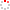NSSC OpenIR  > 空间科学部
 Motion of observed structures calculated from multi-point magnetic field measurements: Application to Cluster Shi, Q. Q.; Shen, C.; Dunlop, M. W.; Pu, Z. Y.; Zong, Q. G.; Liu, Z. X.; Lucek, E.; Balogh, A.; 北京8701信箱 Department 空间天气学国家重点实验室 Source Publication Geophysical Research Letters2006 Volume 33Issue:8Pages:L08109 ISSN 0094-8276 Language 英语 Keyword Art. Orientation Discontinuity Residue Density Abstract A new method is described which calculates the velocity of observed, quasi-stationary structures at every moment in time from multi-point magnetic field measurements. Once the magnetic gradient tensor G=del (B) over right arrow and the time variation of the magnetic field have been estimated at every moment, the velocity can then be determined, in principle, as a function of time. One striking property of this method is that we can calculate the velocity of structures for any dimensionality: for three-dimensional structures, all three components of the velocity vector can be calculated directly; for two-dimensional (or one-dimensional) structures, we can calculate the velocity along two (or one) directions. The advantage of this method is that the velocity is determined instantaneously, point by point through any structure, and so we can see the time variation of the velocity as the spacecraft traverse the structure. In this paper, the feasibility of the method is tested by calculating the motion velocity of a three-dimensional, near cusp structure and a two-dimensional magnetotail current sheet. The results for one-dimensional structures in the magnetopause and cusp boundaries are compared to calculations for the standard techniques for analyzing discontinuities. Indexed By SCI Funding Project 中国科学院空间科学与应用研究中心 Document Type 期刊论文 Identifier http://ir.nssc.ac.cn/handle/122/1040 Collection 空间科学部 Corresponding Author 北京8701信箱 Recommended CitationGB/T 7714 Shi, Q. Q.,Shen, C.,Dunlop, M. W.,et al. Motion of observed structures calculated from multi-point magnetic field measurements: Application to Cluster[J]. Geophysical Research Letters,2006,33(8):L08109. APA Shi, Q. Q..,Shen, C..,Dunlop, M. W..,Pu, Z. Y..,Zong, Q. G..,...&北京8701信箱.(2006).Motion of observed structures calculated from multi-point magnetic field measurements: Application to Cluster.Geophysical Research Letters,33(8),L08109. MLA Shi, Q. Q.,et al."Motion of observed structures calculated from multi-point magnetic field measurements: Application to Cluster".Geophysical Research Letters 33.8(2006):L08109.